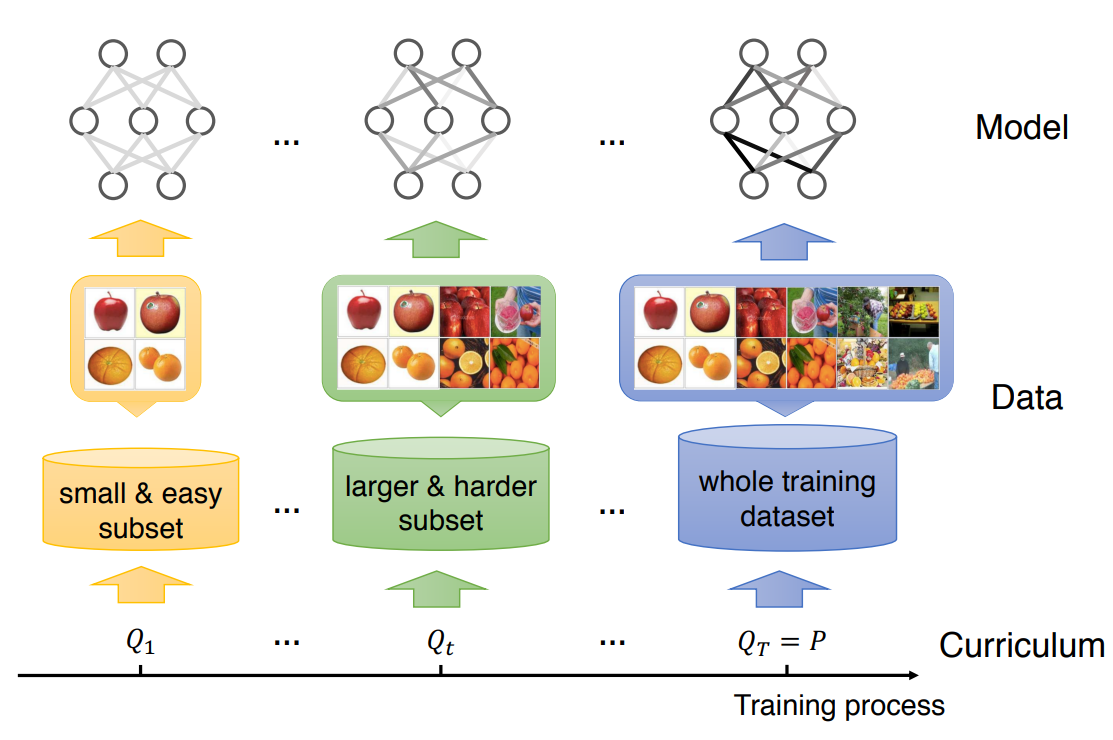# Curriculum learning imitates human learning order from easier classes to harder classes

Last updated on：a year ago

Curriculum learning (CL) is a training strategy that trains a machine learning model from easier data to harder data (with a curriculum), which imitates the meaningful learning order in human curricula.# Introduction

1. Original curriculum learning. A curriculum is a sequence of training criteria over T training steps. Each criterion $Q_t$ is a reweighting of the target training distribution $P(z)$.
Conditions satisfied:
1. The entropy of distributions gradually increases
2. The weight for any example increases
1. Data-level generalized curriculum learning. Discarding all three conditions in definition 1, a curriculum is a sequence of reweighting of target training distribution over T training steps.

2. Generalized curriculum learning. A curriculum is a sequence of training criteria over T training steps. Each criterion $Q_t$ includes the design for all the elements in training a machine learning model.

# Reference

 Wang, X., Chen, Y. and Zhu, W., 2021. A survey on curriculum learning. IEEE Transactions on Pattern Analysis and Machine Intelligence.